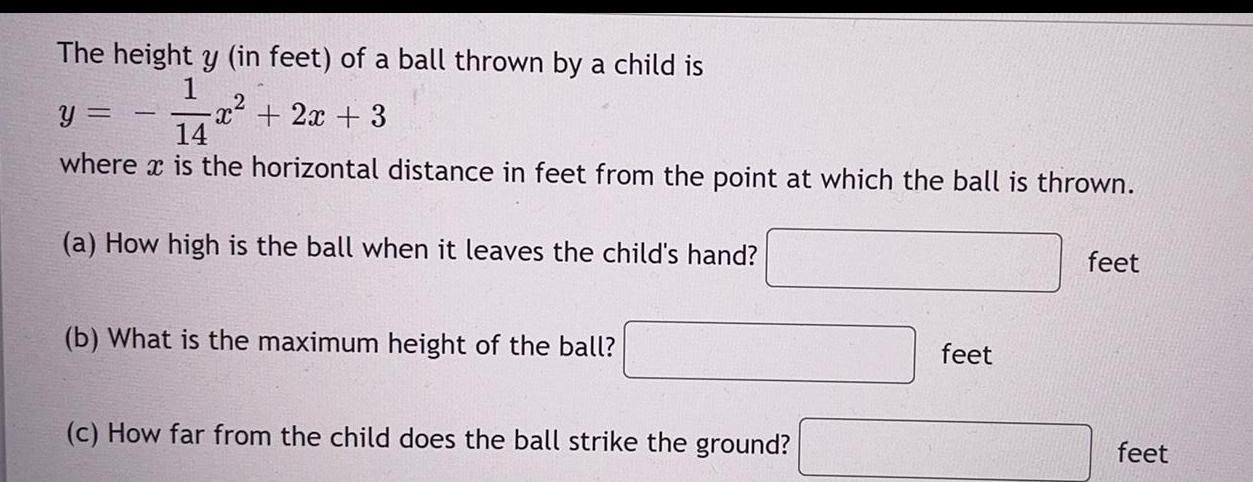Question:

# The height y (in feet) of a ball thrown by a child is

Last updated: 8/26/2022The height y (in feet) of a ball thrown by a child is y=-1/14 x² + 2x + 3 where is the horizontal distance in feet from the point at which the ball is thrown. (a) How high is the ball when it leaves the child's hand? feet (b) What is the maximum height of the ball? feet (c) How far from the child does the ball strike the ground? feet